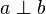# Coprime facts for kids

Kids Encyclopedia Facts

In mathematics, two integers (a and b) are co-prime (or relatively prime) if they share no common factors. This is sometimes written as$a \perp b$. In other words, there is no number, other than 1, that divides both a and b evenly. In which case, the greatest common divisor (GCD, or highest common factor) of these numbers is 1.

As an example, 6 and 35 are coprime, because the factors of 6, 2 and 3, do not divide 35 evenly. On the other hand, 6 and 27 are not coprime, because 3 divides both 6 and 27. Another example is 4 and 5: 4 = 2*2*1; 5 = 5*1 (Prime). The only common factor is 1, so they are coprime.

On the other hand, 10 and 5: 10 = 5*2 5 = 5*1 (Prime). The common factors are 5 and 1, so they are not coprime.

Prime numbers are always coprime to each other.

1. Any two consecutive integers are always coprime.
2. Sum of any two coprime numbers is always coprime to their product.
3. 1 is trivially coprime with all numbers.
4. if out of two numbers, any one number is a prime number while the other number is not a multiple of first one, then both are coprime.
5. This is not applicable to negative numbers

## Related pagesCoprime Facts for Kids. Kiddle Encyclopedia.